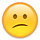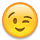# Voltage loss vs ?

OK I am having real difficulty in understanding the "voltage loss" question. I am aware that DC differs from AC thank you Mr. Tesla, but the only apparent difference (limited understanding) is the ability to step up/down the voltage easily through a transformer.
The problem with voltage loss as I currently understand it (subject to change with more information) is having enough voltage to charge a battery at 14. what ever V necessary for that battery, requires that you have enough more than that so that any loss is less than that which allows 14.4V at the battery terminals.
Given the voltage from the panel(s) is in my case 43 volts under load and the controller can handle that, Morningstar MPPT can, and that the output can be regulated to match the voltage the Concord AGM battery requires at the battery terminals adjusting for the voltage loss in the wire from the charge controller.
Is there a reason to use more than a 10ga wire?

• Banned Posts: 17,615 ✭✭
Re: Voltage loss vs ?

It depends on how long the wire run is (both ways). The longer the run, the more resistance and the greater the Voltage drop. Larger wire has less resistance per foot than smaller wire.

If you keep your longest run from the 48 Volt (interesting Vmp) array to the MPPT controller and the lines from the controller to the battery short this is the best case.

But get the distances and do the calcs; it's very important on "12 Volt" systems.
• Re: Voltage loss vs ?

Voltage drop is a function of at least four factors:
• Volts
• Amp
• Wiresize (I'm assuming copper and ignoring aluminum)
• Distance

With low voltage, you lose a significant percentage of the voltage in a much shorter length than most people expect.

Using the "Conductor Maximum Length Voltage Drop Calculator " calculator at http://www.electrician2.com/calculators/vd_calculator.html, I find that with #12 copper, 14 volts, 10 amps, at 7.09 feet there is a 2% voltage drop. Increasing the wire size to #8 increases the 2% voltage drop maximum to 17.92 feet.

However, 28 volts at 5 amps has a 2% voltage drop maximum of 28.35 feet with #12 wire.

Since solar electric is relatively expensive, it makes sense to design the system carefully so you don't lose electricity in the wires.

Keith
• Solar Expert Posts: 10,300 ✭✭✭✭
Re: Voltage loss vs ?

i'm not sure if shadowcatcher really was talking of just the wires and their losses as he references to battery charging voltages and if i miss my guess here he may want to know why the voltage has to be higher to actually induce a charge to the battery. it is for similar reasons though because every battery has an internal resistance. to allow more current flow the only way to do that in a battery is to increase the voltage because one cannot change the resistance in the battery. this is why higher voltages are needed just to induce a charge, but controllers also need a bit of leeway here for them to properly operate too.

generally comparative examples to show the higher currents can be yielded from higher voltages with a steady resistance of say .01 ohms (this is only illustrative here for an example) using ohm's law of i(current) = e(volts) / r(resistance):

.5v/.01ohms=50a
1v/.01ohms=100a
2v/.01ohms=200a

note that the voltage listed above would be the difference between that of the battery and of the charging source.

now wires are going to a battery have resistance, but the batteries themselves have a resistance and is known as internal resistance and this gets added to the resistive losses elsewhere like from your wires. resistance is, as its name implies, the opposite of conduction and it's conduction you want.

even if this was not part of the original question, it doesn't hurt for getting this aspect of understanding.
Re: Voltage loss vs ?

And to and my \$1.99.... You can figure out the costs of power losses pretty easily... Assume your off-grid power costs you around \$1-\$2+ per kWH (say \$1.50 for this example).

Say system is 1,000 watts of panels, 4 hours of sun average per day, and 1% vs 3% wiring drop (about 4.5 awg heavier wire, or 3x more copper):
• 1kW panels * 4 hours per day sun * 0.77 array derating * 365 days = 1,124 kWH per year
• 1,124 kWH * 0.01 = 11 kWH lost at 1% wiring drop
• 1,124 kWH * 0.01 = 34 kWH lost at 1% wiring drop
• 34 - 11 = ~23 kWH per year difference between 1% and 3% wiring
• 23 kWH * 20 year life * \$1.50 per kWH est. power costs = \$690 worth of energy over 20 years...
So, a very simple model (no interest, no present value / future worth formula, assumes 100% of solar power collected is used, etc.) would give you a ~\$690 break even cost between a 1% and a 3% cost difference between designs over 20 years.

Pick your numbers.-Bill
Near San Francisco California: 3.5kWatt Grid Tied Solar power system+small backup genset